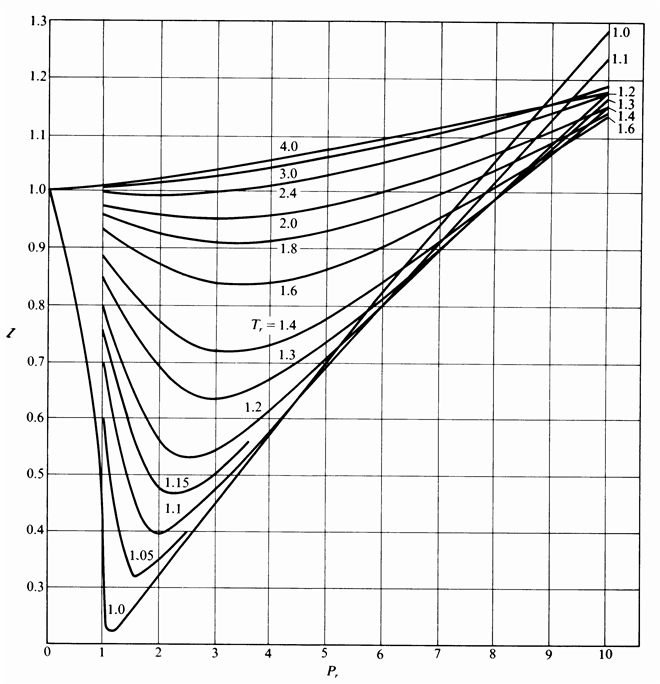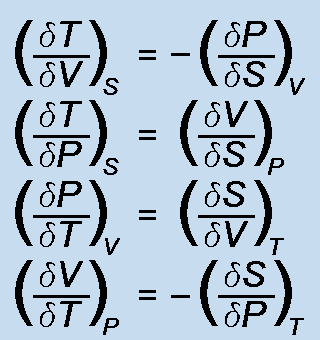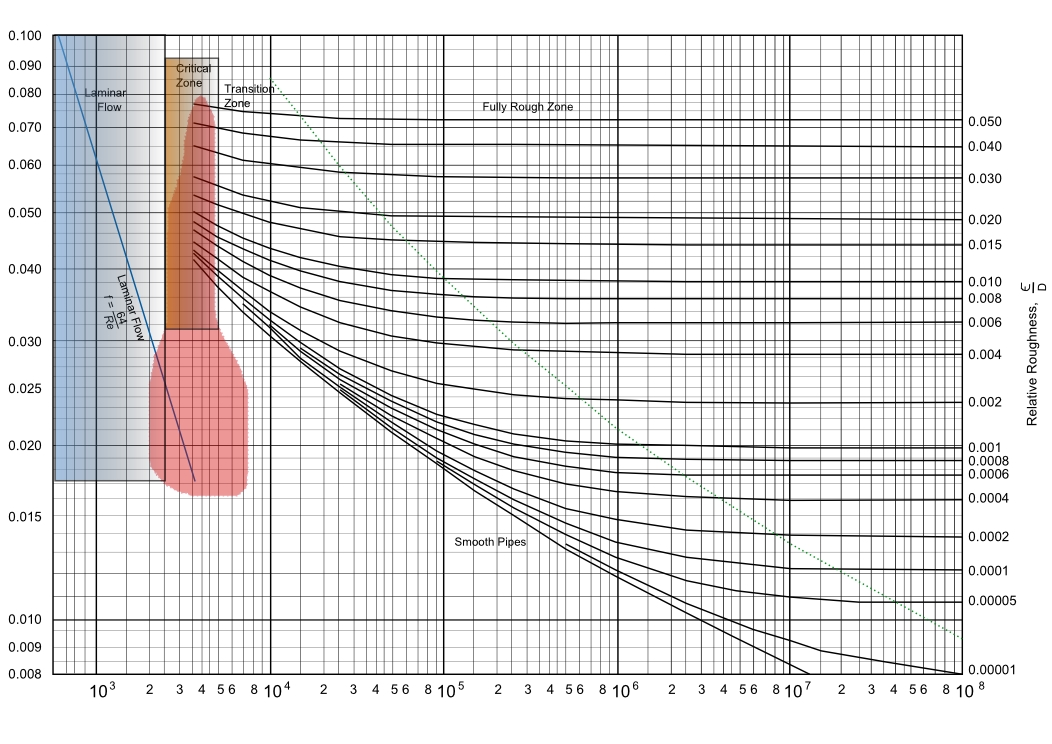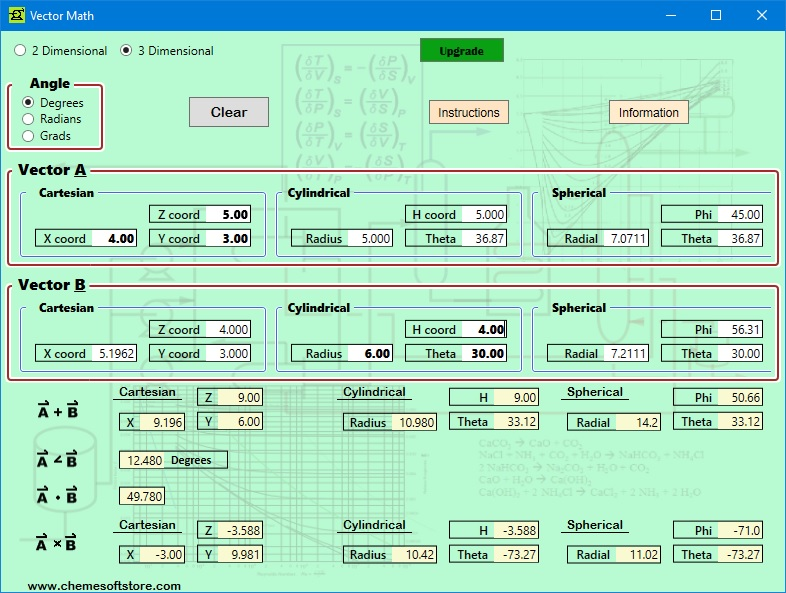### ChemE Soft StoreCaCO3 -> CaO + CO2
NaCl + NH3 + CO2 + H2O -> NaHCO3 + NH4Cl
2 NaHCO3 -> Na2 CO3 + H2O + CO2
CaO + H2 O -> Ca(OH)2
Ca(OH)2 + 2 NH4 Cl -> CaCl2 + 2 NH3 + 2 H2O

## Vector Math

Vector math includes three dimensional conversion between cartesian, cylindrical, and spherical co-ordinate systems. Also addition, angle of intersection, dot and cross product calculations.#### Features:

• Both two and three dimensions can be solved
• Input vector coordinates are highlighted in BOLD font with calculated values shown in other boxes
• Different angle units can be used
• Information on vector math and the different coordinate systems is presented
• Instructions for the software application is presented